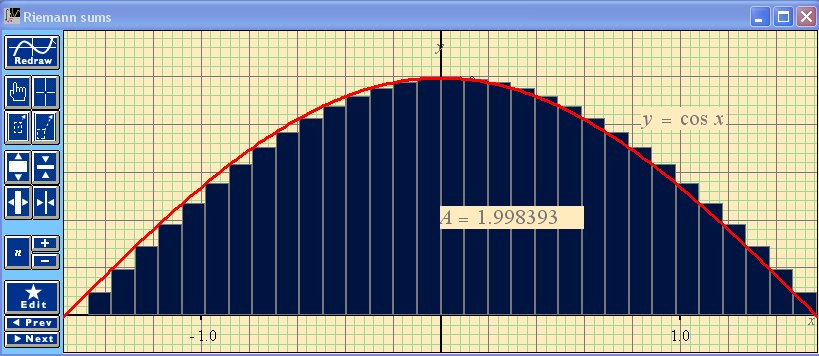# Help With Math: Purveyors of MathXpert

-->This graph shows a typical Riemann sum, approximating the area under a curve as a sum of areas of thin rectangles. With a click of a button (in MathXpert, not on this web page) you can change the number of rectangles. The total area of the rectangles is displayed. The Edit button gives you the ability to specify different kinds of Riemann sums (centered, left, or right). MathXpert also can make similar graphs using the trapezoid rule and Simpson's rule.